Viewing posts from August, 2007

## The Smoking Xis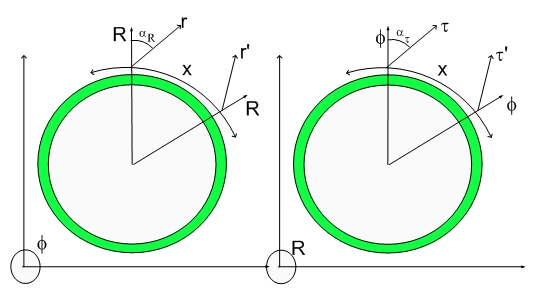THE SMOKING XIS

Coming down the pipe are the XIS. Below are the assignment for XI Zero and XI Minus: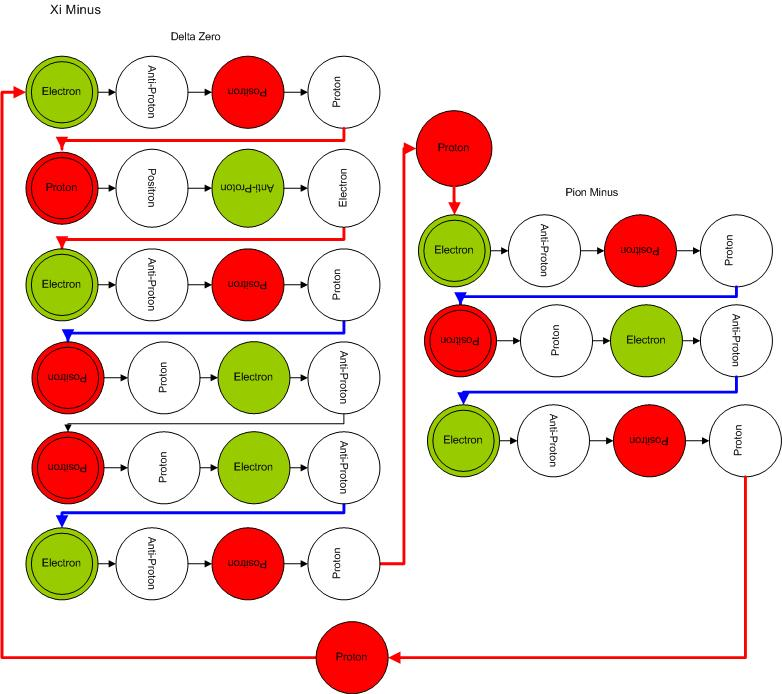which decays into Lambda Zero plus a Pion Minus...:) (remember that Lambda Zero is just another spin configuration of Delta Zero)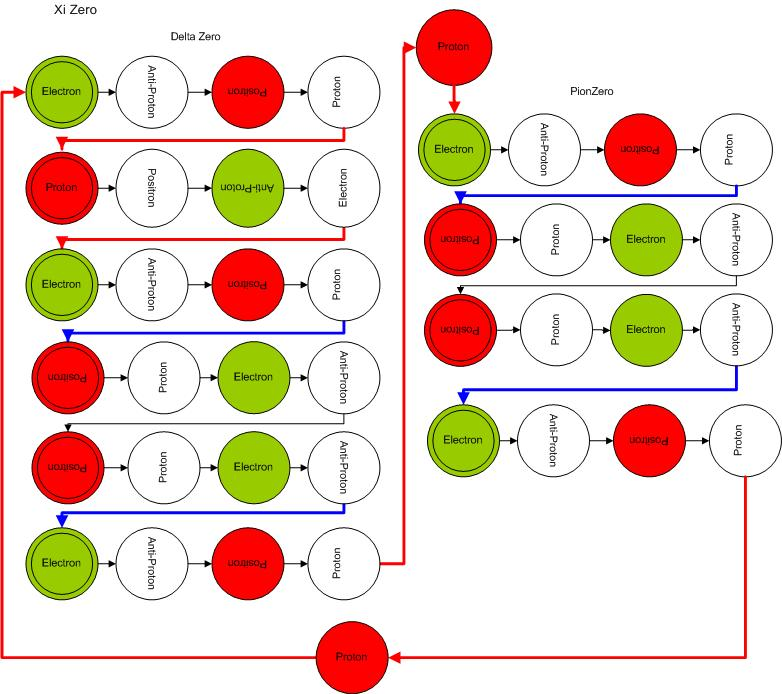## The KaonsThe Kaons

The Kaons is a detour. Up to know we only analysed particles that contained Electron-Neutrinos (Electron-Proton-Electron transmutation transitions) or Muon-Neutrinos (Electron-Positron-Electron transmutation transitions)

The Kaons will involve the last known neutrino: The Tau Neutrino.

Let's start with the Kaon Minus assignment: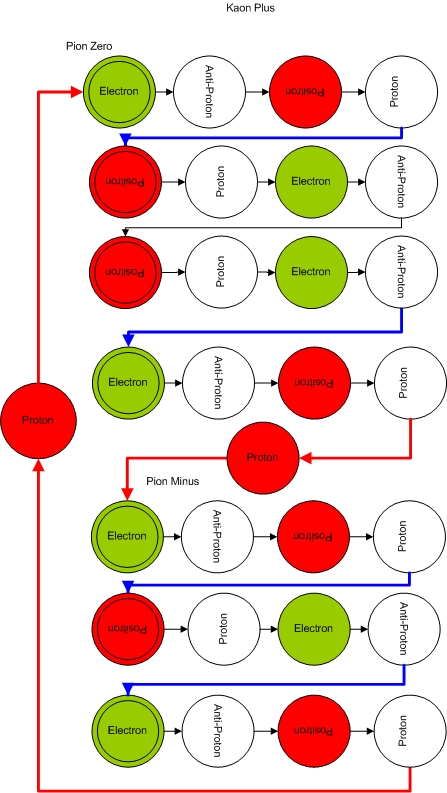which decays into a Pion Minus plus a Pion Zero.

## The Twisted Deltas - The Puzzling Delta Plus Plus## The Twisted Deltas - The Great Delta ZeroThe Twisted Deltas - The Great Delta Zero

The next Delta will blow your mind away...:) it becomes more complex as time goes by...:) I can see your Brain gears moving already...:)Delta Zero is a step above in terms of complexity both structurally and in terms of the dissociation dynamics.
Below is its assignment. The assignment is based upon the decay pathway: DeltaZero -> Neutron plus PionZeroA simple rearrangement of red and blue lines yields the diagram below: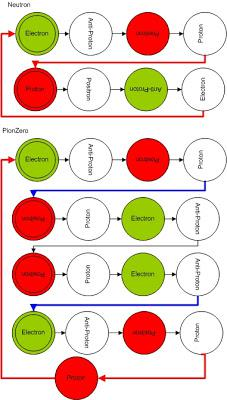The other decay pathways is more complex: DeltaZero -> Proton + Pion Minus.

The first step in this pathway is shown below: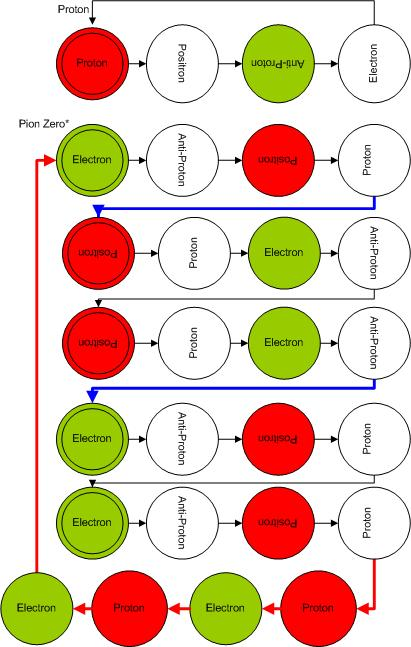This shows the Proton detachment but it is still unclear how the other moiety can correspond to a Pion Minus...:) The first step in the simplification is to eliminate the Electron-Proton spin 1/2 followed by an Proton-Electron spin -1/2 transmutation, leaving the basic Pion Minus plus an electron and a positron extras.

To understand the next decaying steps, one should think about these multichords in a multimeric fashion. This initial stage is pentameric. The state of the products can be understood from the diagram below: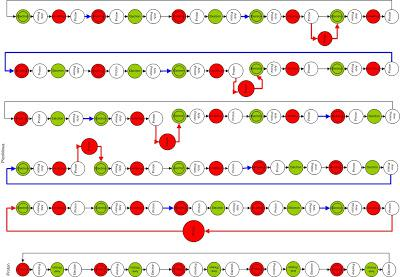Notice the five concurrent multichords associated with this excited state Pion Minus. This means just after Proton detachments, one is left with a pentameric moiety. This means that there will be a positron and an electron with opposing spins facing each other in the 3D Universe. The metric displacement volumes associated with both adds up to zero and that means that there will be no loss of 3D Volume (3D Mass or Energy) if a Positron and its counterparty electron are eliminated from the multichord coherence. The resulting trimeric moiety is what we call Pion Minus and it is shown below: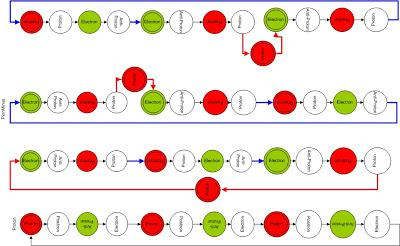which is the same as :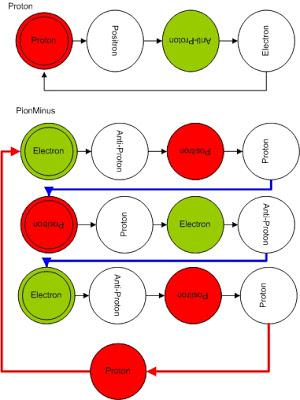in our short hand representation.

Remember that a multichord particle will have an extra degree of freedom (the 3D moment of inertia of the "nuclear" particle). It pseudo-rotate at a very specific angle at each de Broglie step of the Shock Wave Universe expansion.

This extra degree of freedom permits the positron electron anihilation without change in the 3D Mass or energy.

I hope you are having fun...:)

Sigma Zero decays into Lambda Zero plus Gamma Ray, thus it should contain and extra electron-positron pair responsible by the Gamma Ray.

## Pion Zero - Majorama CoherencePion Zero - Majorama Coherence

Theoreticians make a big fuss of Majorama particles....:) It turned out that their speculation was correct (within my model) and that Pion Zero is its own anti-Particle...:)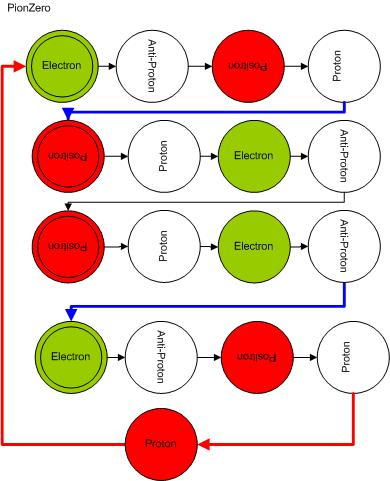It should be clear that one can rearrange the coherence such that the electrons go into the interior of the multichord coherence and that Electron-Proton-Electron is the same as Positron-AntiProton-Positro transmutation transition.